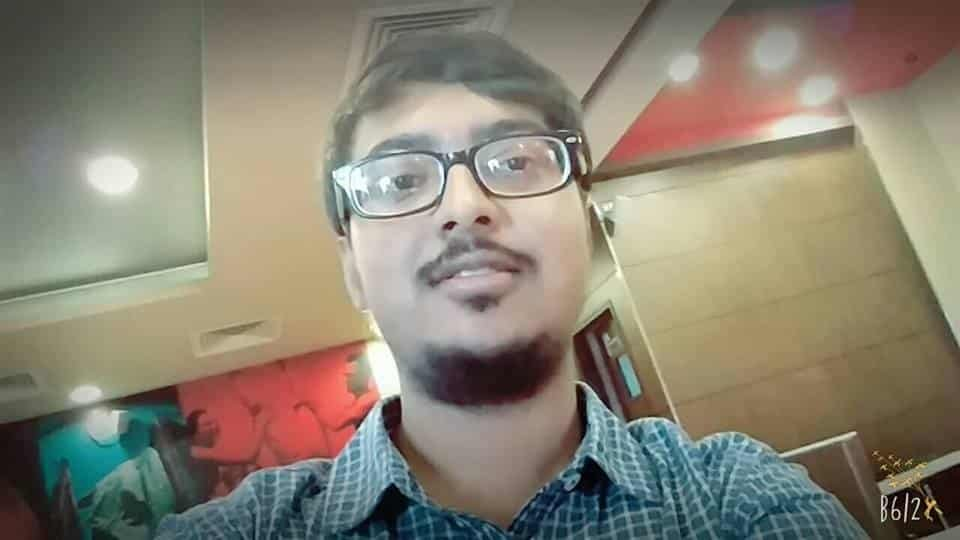Categories

# CMI Entrance 2019 – Answer Key, Sequential Hints

CMI (Chennai Mathematical Institute) Entrance 2019, Sequential hints, answer key, solutions.

#### Please submit problems in the comment section. Try to make the statement as close to the actual statement as possible.

This post contains questions from Chennai Mathematical Institute, CMI Entrance 2019, Sequential hints, answer key, solutions. Our team will upload the solutions. Problems are contributed by students.

## Problems

1. Suppose $\frac{8^x + 27^x}{12^x + 18 ^x} = 7/6$. Find x.

2. There is a parallelogram ABCD. O is any point in the interior such that angle AOB + angle DOC = 180. Prove that angle ODC= angle OBC

3. Find the number of natural number n for which $n^6+n^4+1$ is a perfect square

4. $\phi_k (m) = \phi_1 (\phi_{k-1} (m))$  and $\phi_1 (m)$ is number of divisors of m . Find k for which $\phi_k (2019^2019) =2$

5. If there exists a calculator with 12 buttons, 10 being the buttons for the digits and A and B being two buttons being processes where if n is displayed on the calculator if A is pressed it increases the displayed number by 1 and if B is pressed it multiplies n by 2 hence 2n. Hence find the minimum number of moves to get 260 from 1

6. Find the sum 1+111+11111+1111111+…..1….111(2k+1) ones

 1 2 3 4 5 6 7 8 9 10 11 12 13 14 15 16 17 18 19 20 21 22 23 24 25 26 27 28 29 30

## Faculty Team (for this section)#### Ashani Dasgupta

Founder – Faculty at Cheenta. Pursuing Ph.D. at University of Wisconsin, Milwaukee. USA Research Interest: Geometric Group Theory#### Srijit Mukherjee

Faculty – Admin at Cheenta Pursuing B.Stat at Indian Statistical Institute, India#### Writabrata Bhattacharya

Faculty at Cheenta Pursuing B.Sc. Math at Chennai Mathematical Institute, India Research Interest – Algebraic Geometry#### Ishan Sengupta

Associate Faculty at Cheenta

Pursuing B.Stat from Indian Statistical Institute, India## By Dr. Ashani Dasgupta

Ph.D. in Mathematics, University of Wisconsin, Milwaukee, United States.

Research Interest: Geometric Group Theory, Relatively Hyperbolic Groups.

Founder, Cheenta

## 11 replies on “CMI Entrance 2019 – Answer Key, Sequential Hints”Ankush Agarwalsays:

Evaluate the integral

Integral from 0 to e^x of log(t)cos^4(t)dtSanjana Hukkerisays:

Part B of the paper :

Integral-> 0 to infinity
dx/(1+x^2)^(m+1)

Thank youDarsansays:

Probability of two rooks attacking each other in a 8*8 chessboardSupriyo Haldersays:

In a 8×8 chessboard two different squares are chosen at random.If two rooks are placed in these two squares , what is the probability that they will attack each other.(Remember that the rooks can move horizontally or vertically from their position)TRISHAN MONDALsays:

think that one rook is at corner . now it has actually 14 options where another rook can be placed
if u take it forword one row it has actually 13 options . note that u can take it upto 4 row.

here (14+13+12+11) case arose .

now start it to move column wise u get same result

now take it up (1,1) position it also has (14 ) option and then it redused to 13,12,11 respectively

it can move in ( 4*4) squere

n(a) = 16(50)

n(s) = 64c2 = 63*32

p(a) = 25/63Supriyo Haldersays:

You have a land in side of a river which is flowing straight.You want to take a rectangular shape of the land taking the bank of the river as one of its side.So, you can fence only three sides of the land.If you have only 60m of fence then what is the maximum area of your land?

Thank you.Supriyo Haldersays:

Consider the function f:R×R–>R defined by f((a,b))=lim_n–>inf (ln(e^(na)+e^(nb)))/n.For each of the following statements state sequentially whether they are true or false (write T for true and F for false,your answer should be in the form like TTF)
1.f(0,x)=x for all x>=0
2.f is not onto on R
3.For every b the function f(x,b) is continuous on R
4.For every a the function f(a,x) is differentiable on R

Thank youSuryasays: